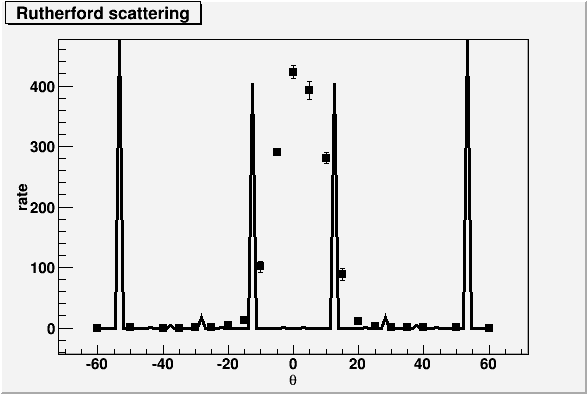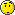# Problem with rutherford fit

I have to fit the data of a rutherford scattering, but the fit is very bad. Changing the initial fit parameters, the situation does not improve.
I have attached the minimum running script I used and the graph that this script generates.
I use ROOT 5.18/00.
Can someone help me, to have a good fit?

Thanksrutherford.C (1.72 KB)

You must set some original values for your 2 parameters.

Rene

I tried, putting reasonable values. But the result is the same.

so it means that the model of your function is wrong for fitting your data.

Rene…these are data of a rutherford scattering experiment, and the rutherford cross section is ~A*sin(x/2)^(-4) where x is the angle. The formula seems to be correct.

hmmm, this fitting problem is not well defined:

1. you plot something as a function of theta but the formula clearly
requires a theta/2 . So either cleanup your table or your formula
2. more scarry is that your Rutherford formula has a pole at theta=0.
your data has not , because you made a measurement with a
finite aperature . So your formula should be a convolution between
Rutherford and some acceptance function (take a gauss for instance)
3. Several data points have ey = 0 ???, so you should use a counting
statistic option (Poisson). On the other hand, I notice that the y-error
is not the sqrt(y)?

Eddy